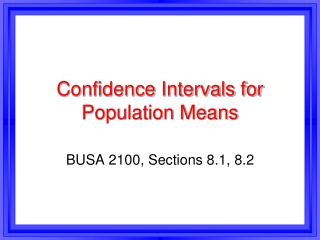DownloadDownload PresentationConfidence Intervals for Population Means

# Confidence Intervals for Population Means

Télécharger la présentation## Confidence Intervals for Population Means

- - - - - - - - - - - - - - - - - - - - - - - - - - - E N D - - - - - - - - - - - - - - - - - - - - - - - - - - -
##### Presentation Transcript

1. Confidence Intervals for Population Means BUSA 2100, Sections 8.1, 8.2

2. Estimation Process • Point estimates are single numbers, e.g. X-bar = \$35,000. • Point estimates should be close to the true population mean (or proportion), but are almost never exactly equal. • So we will use interval estimates, known as confidence intervals. • Confidence intervals are a range of numbers, e.g. \$30,000 to \$40,000.

3. Confidence Intervals • Two conditions for confidence intervals: • 1. We want them to be narrow. • For example, the interval \$15,000 to \$55,000 is not a useful estimate for the mean salary of a firm’s employees. (too wide). • But the interval \$34,000 - \$36,000 is useful. • 2. We want them to have a highprobability (usually >= 90%) of containing the population mean (or proportion).

4. Confidence Levels • The probability that a confidence inter-val contains the population mean or proportion is the confidence level. • The most common confidence levels are 90%, 95%, and 99%. • In a normal distribution, how many std. deviations away from the mean do we need to go to include 95% of the items?

5. Accountant Incomes Example • Example: A random sample of 64 accountants in Georgia is selected and their annual incomes are recorded. • The sample mean is \$55,000 and the sample std. deviation is \$4,000. • Find a 90% confidence interval for the population mean annual income.

6. Incomes Example, Page 4 • Part b : Find a 95% confidence interval.

7. Incomes Example, Page 5 • Part c: Find a 99% confidence interval if the sample size is 400.

8. Confidence Intervals for Small Samples or Sigma Unknown • For sample sizes < 100 or when sigma is unknown, we use the t-distribution instead of the normal curve table. (In some situa-tions, the normal curve table can still be used for sample sizes much less than 100.) • Same process, except use t-values instead of z-values. • t-values depend on the sample size, or degrees of freedom.

9. The t-Distribution • The number of degrees of freedom is 1 less than the sample size; df = n - 1 . • The t-table is based upon areas in 1 tail.

10. Sample Size and t-values • Look up t-values for various confidence levels and sample sizes. • For small n and df values, the t-values are very large. This creates wide confi-ence intervals with poor accuracy.

11. Confidence Interval Formula for Means, Small Sample Size • Ex.: For a sample of 15 trainees, the sample mean training time is 26 hours & the sample std. deviation is 4.2 hours. • Find a 99% C.I. for the population mean training time.